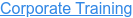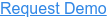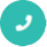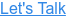#### 15+ Categories. 500+ IT Courses to Learn.# R Interview Questions And Answers

by Mohammed, on Mar 28, 2018 12:57:40 PM## Q1. What is R?

Ans: R is a language and environment for statistical computing and graphics. It is an open source programming language. R provides a wide variety of statistical and graphical techniques and is highly extensible. Data miners use it for developing statistical software and data analysis. One of the R’s strengths is the ease with which well-designed publication-quality plots can be produced, including mathematical symbols and formula where needed. R is available as Free Software under the terms of the Free Software Foundation’s GNU General Public License in source code form. It compiles and runs on a wide variety of UNIX platforms and similar systems (including FreeBSD and Linux), Windows and MacOS. The R command line interface(CLI) consist of a prompt, usually the > character.

## Q2. Is R is a slow language?

Ans:

• R programs can be slow, but well-written R programs are usually fast enough.
• Speed was not the primary design criteria.
• Designed to make programming easier.
• Slow programs often a result of bad programming practices or not
understanding how R works.
• There are various options for calling C or C++ functions from R

## Q3. List  the different data structures in R langauage?

Ans: R contains primarily the following data structures:

• Vector
• Matrix
• Array
• List
• Data frame
• Factor

The first three data types (vector, matrix, array) are homogeneous in behavior. It means all contents must be of the same type. The fourth and fifth data types (list, data frame)are heterogeneous in behavior. It implies they allow different types. And the factor data type is used to store categorical variable.

## Q4. What are programming features of R?

Ans:

• Packages are part of R programming. Hence, they are useful in collecting sets of R functions into
a single unit.
• R’s programming features include database input, exporting data, viewing data, variable labels,
missing data, etc.
• R is an interpreted language. So we can access it through command line interpreter.
• R supports matrix arithmetic.
• R supports procedural programming with functions and object-oriented programming with
generic functions. Procedural programming includes procedure, records, modules, and
procedure calls while object-oriented programming language includes class, objects, and
functions.

## Q5. What are R Functions?

Ans: A function is a piece of code written to carry out a specified task. Thus it can or can’t accept arguments or parameters and it can or can’t return one or more values. In R, functions are objects in their own right. Hence, we can work with them exactly the same way we work with any other type of object.

## Q6. What are the different data objects in R?

Ans: Data Objects in R are listed below:

• vectors
• lists
• arrays
• matrices
• data frames
• tables

## Q7. What makes a variable name valid in R?

Ans: Variable name starts with an alphabet letter
Variable consisting of number, dot, and underscore

## Q8. What is R Base package?

Ans: R Base package basically provides input/output functionality and arithmetic calculation functionality.R Base package is automatically installed at the time of R Programming Environment setup.

## Q9. What is Predictive analysis process in R?

Ans:

• Define Project: It includes Project outcomes, business objectives, deliverables, scoping of the effects.
• Data Collection: For predictive analysis, it collects data from different sources to analysis. Thus it provides a complete view of customer interactions.
• Data Analysis: It is the process of cleaning, transforming, inspecting and modeling data. The goal of this process is to discover useful information.
• Statistics: This process enables to confirm the assumptions. Hence it uses the assumption to test using a statistical model.
• Modeling: An accurate predictive model about future is been created using predictive modeling. There are also options to choose the best model.
• Deployment: To deploy the analytical results into everyday decision-making.
• Model Monitoring: To ensure that it is providing an expected result, we have to manage model.

## Q10. What are the various sorting algorithms in R?

Ans: Major five sorting algorithms:

1. Bubble Sort
2. Selection Sort
3. Merge Sort
4. Quick Sort
5. Bucket Sort

## Q11. What are the applications of R?

Ans:

• Many data analysts and research programmers use R because R is the most prevalent language. Hence, R is used as a fundamental tool for finance.
• Many quantitative analysts use R as their programming tool. Hence, R helps in data importing and cleaning, depending on what manner of strategy you are using on.
• R is best for data Science because it gives a broad variety of statistics. In addition, R provides the environment for statistical computing and design. Rather R considers as an alternate execution of S.

## Q12. What are various ways to write loop in R

Ans: There are primarily three ways to write loop in R

1. For Loop
2. While Loop
3. Apply Family of Functions such as Apply, Lapply, Sapply etc

## Q13. What is Visualization in R?

Ans: Visualization is any technique for creating images, diagrams, or animations to communicate a message. Visualization through visual imagery has been an effective way to communicate both abstract and concrete ideas since the dawn of humanity.

## Q14. What are statistical and programming features of R?

Ans: Statistical Features:

• Basic Statistics: Mean, variance, median.
• Static graphics: Basic plots, graphic maps.
• Probability distributions: Beta, Binomial.

Programming Features:

• Distributed Computing: Distributed computing is an open source, high-performance platform for the R language. It splits tasks between multiple processing nodes to reduce execution time and analyze large datasets.
• R packages: R packages are a collection of R functions, compiled code and sample data. By default, R installs a set of packages during installation.

## Q15. What are Generic Functions in R?

Ans: R has three object-oriented (OO) systems: [[S3]], [[S4]] and [[R5]]. … A method is a function associated with a particular type of object. S3 implements a style of object-oriented programming called generic-function OO

Ans:

• Mean
• Median
• Distribution
• Covariance
• Regression

## Q17. What is R’s C interface?

Ans: R’s source code is a powerful technique for Improving Programming skills. But, many base R function was already written in C. It is been used to figure out how those functions work. All functions in R defined with the prefix Rf_ or R_.
Outline of Rs C interface

• Input Validations talks about itself so that C function doesn’t crash R.
• C data Structures shows how to translate data structure names from R to C.
• Creating and modifying vectors teaches how to create, change, and make vectors in C.
• Calling C defines the basics of creating. It also defines the functions with the inline package.

## Q18. What is reshaping of data in R?

Ans: In R Programming, reshaping of data is the conversion of one data object into other data object.

## Q19.  How is R used in logistic regression?

Ans: In R Programming, glm() method is used to create logistic regression and logistic regression works with measuring the probability of a binary response variable.

## Q20. How can you load and use CSV files in R?

Ans: In R Programming language, read.cvs method is used to load CSV files.